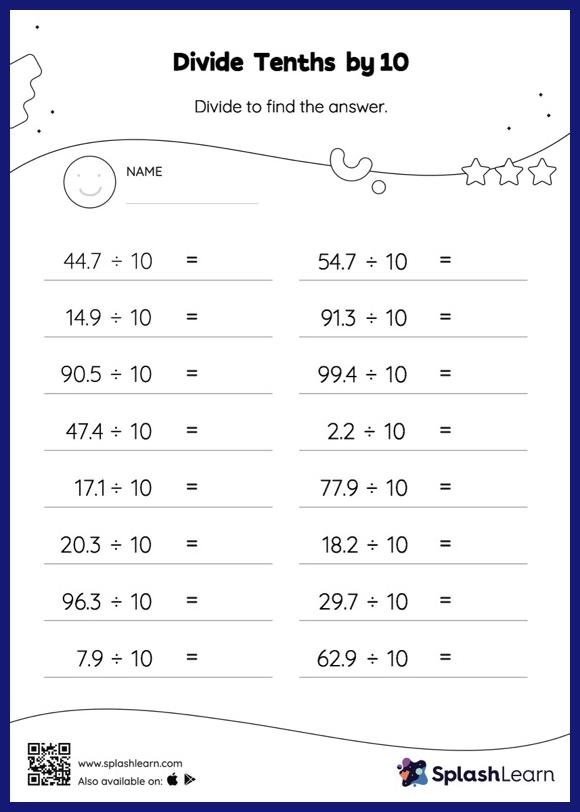# Divide Tenths by 10: Horizontal Division Worksheet

Home > Divide Tenths by 10: Horizontal DivisionThis divide tenths by 10 worksheet consists of a set of questions on division, which help students develop fluency. Dividing a number by 10 moves each digit one place to the right. Divide tenths by 10 worksheet provides plenty of opportunities to practice this concept. In this worksheet, students practice solving problems written in the horizontal format. How numbers are laid out in a problem affects the method a student employs to solve it. Therefore to develop actual fluency and mastery of multiple strategies, students must practice different formats.